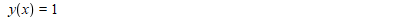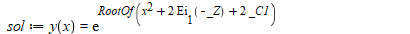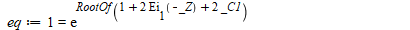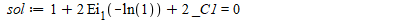## Maple Animation of 150 Days of Corona in the US ...

by: Maple 2020

This is an animation of the spread of the COVID-19 over the U.S. in the first 150 days.  It was created in Maple 2020, making extensive use of DataFrames.

The animation of 150 Day history includes COVID-19 data published by the NY Times and geographic data assembled from other sources. Each cylinder represents a county or in two special cases New York City and Kansas City. The cross-sectional area of each cylinder is the area in square miles of the corresponding county. The height of each cylinder is on a logarithmic scale (in particular it is 100*log base 2 of the case count for the county. The argument of the logarithm function is the number of cases per county divided by the are in square miles-so an areal density.  Using a logarithmic scale facilitates showing super high density areas (e.g., NYC) along with lower density areas.  The heights are scaled by a prefactor of 100 for visualization.

## need code, its very urgent...

Hello all

could anyone tell how to solve following nonliner equations numerically.

f '''' - c1(g'') + R(f ' f '' - f f ''' )=0

g'' + c2(f '' -2g) -c3(f g' - f ' g)=0

f ' (-1)=0,   f ' (1)=0,   f(-1)=1-A, F(1) =1, g(-1)=0, g(1)=0

c1=3.2, c2=3.3, c3=3.4, R= -10 and A=1.6 are constants.

## Why integration of dirac delta with argument sin i...

Hello,

I am trying to calculate an integral involving dirac delta as given below

```int(Dirac(sin(x)),x=-3/2*Pi..3/2*Pi)
```

However, Maple returns the integral unevaluated asHow can I get it evaluated? the result should be 3 here.

## Physics package update...

Hello each and everyone,

I have a problem with updating the Physics Package. (At the moment I run version 709)

But when I try to update to version 710 I have the following error (see the screenshot)

I don't know what's happening.

Kind regards to all.

Jean-Michel## how did Maple obtain this ode solution?...

I can't figure out how Maple obtained this solution and looking for some ideas to try.

It is first order non-linear ode in y(x), which is separable.

```ode:=diff(y(x),x)=x*ln(y(x));
dsolve([ode,y(1)=1],y(x))
```But the general solution is

`sol:=dsolve(ode)`Setting up manually an equation using the given condition in order to solve for _C1, produces no solution.

```eq:=subs([y(x)=1,x=1],sol);
solve(eq,_C1)
```Warning, solutions may have been lost

Also

`coulditbe(exp(RootOf(1 + 2*Ei(1, -_Z) + 2*_C1))=1)`

FAIL

So how did Maple solve for the constant of integration which results in particular solution y(x)=1 that is supposed to satisfy the condition y(1)=1?

It is clear that y(x)=1 satisfies the ODE itself. But I am asking about how it also satisfies y(1)=1

(odetst says it does satisfy the ODE and condition as well. So Maple must have done something very smart under the cover)

Next I tried

```ode:=diff(y(x),x)=x*ln(y(x));
sol:=dsolve(ode,y(x));
sol:=DEtools:-remove_RootOf(sol);
sol:=subs([y(x)=1,x=1],sol)
```And now

```solve(sol,_C1)
```

Error, (in Ei) numeric exception: division by zero

Just wondering how did Maple decide that y(x)=1 satisfies y(1)=1? I do not see it.

Using Maple 2020.1. But same result on Maple 2019

## How to display Trigonométric values of the Explo...

Hi,

How to display only trigonometric values ( like 0,Pi/6,Pi/3..)  of the parameter alpha in the Explore Command?

Thanks

ROTATIONConiquesEllipseAnimation.mw

## Ellipse Equation ( rotation)...

Hi ,

i want to find simple equation of rotate Ellipse  ( El1,EL2,El3 in the  worksheet), with calssic formulaIdeas? Thanks

QuestionConiqueRotation.mw

## Find the focus of an ellipse with a tangential gen...

Etude d'un cas particulier a := 5: b := 7: k := 9: A := [a, 0]: B := [0, b]: #A et B fixes P := [t, 0]: Q := [0, k/t]:#P et Q 2 points mobiles cir := -a*x-b*y+x^2+y^2 = 0: sol := solve(subs(y = 5, cir), x): cen := [solve(diff(cir, x)), solve(diff(cir, y))]: x0 := sol: y0 := 5: M := [x0, y0]: R := sqrt(cen^2+cen^2): beta := arctan(diff(solve(EQ(M, cen), y), x)): Recherche des valeurs de t pour que les 2 droites soient perpendiculaires eq := t^2*(y0-b)+t*(a*b-a*y0+b*x0-k)-x0*(a*b-k) = 0; sol := solve(eq, t); t := sol; tp := sol; P1 := [t, 0]; Q1 := [0, k/t]; PQ1 := simplify(x*(-a*b+b*t+k)+y*t*(t-a)-t*(-a*b+b*t+k)) = 0:#1ere tangente PQ2 := simplify(x*(-a*b+b*tp+k)+y*tp*(tp-a)-tp*(-a*b+b*tp+k)) = 0:#2ième tangente P2 := [tp, 0]; Q2 := [0, k/tp]; CIR := implicitplot(cir, x = -4 .. 8, y = -4 .. 12, color = red); Fig := proc (alpha) local Dr1, DR1, Dr2, DR2, N, u0, v0, Po, t, tp, sol; global a, b, k, cen, R; u0 := cen+R*cos(alpha); v0 := cen+R*sin(alpha); N := [u0, v0]; sol := solve(t^2*(v0-b)+t*(b*u0-a*v0+a*b-k)-u0*(a*b-k) = 0, t); t := sol; tp := sol; Dr1 := simplify(x*(-a*b+b*t+k)+y*t*(t-a)-t*(-a*b+b*t+k)) = 0; DR1 := implicitplot(Dr1, x = -4 .. 8, y = -4 .. 12, color = brown); Dr2 := simplify(x*(-a*b+b*tp+k)+y*tp*(tp-a)-tp*(-a*b+b*tp+k)) = 0; DR2 := implicitplot(Dr2, x = -4 .. 8, y = -4 .. 12, color = pink); Po := pointplot([N[]], symbol = solidcircle, color = [black], symbolsize = 8); display([Po, DR1, DR2]) end proc; DrPQ1 := implicitplot(PQ1, x = -4 .. 22, y = -4 .. 12, color = blue); DrPQ2 := implicitplot(PQ2, x = -4 .. 22, y = -4 .. 12, color = blue); Points := pointplot([A[], B[], M[], P1[], P2[], Q1[], Q2[], cen[]], symbol = solidcircle, color = [green], symbolsize = 10); T := plots:-textplot([[A[], "A"], [B[], "B"], [M[], "M"], [P1[], "P1"], [P2[], "P2"], [Q1[], "Q1"], [Q2[], "Q2"], [cen[], "cen"]], font = [times, 10], align = {below, left}); n := 19; display([seq(Fig(2*i*Pi/n), i = 0 .. n), Fig(beta), CIR, DrPQ1, DrPQ2, Points, T], scaling = constrained, size = [500, 500]); I would find out the focus of the ellipse. Thank you.

## Why did Maple miss the multiplication symbol but ...

Theoretically, if the multiplication sign  is missed Maple needs to give reminders or warnings.But the following is not the case, why？I am surprised its output.

x:=1
x := 1
x(2+1)Actually, I want to enter x*(2+1)

1
x(sin(y))Actually, I want to enter x*(sin(y))
1

## Error that should not really occur ...

I am a little confused by why this error occurs in the second line and not the first, as well as the weird details specified in it. I don't know if the commands that are being called are inbuilt or not, but it is a safe bet that they will be. thankyou.

 >>>## "evalf" output depends upon style of input?...

In preparing to sample problems, I came across this difference in an output depending upon the input type: 2d Input vs. Maple Input. Is there a typo on my part?

 >(1)

Very happy with the output of the following line:

 >(2)

But I'm confused about the output of the next line. Is it a limit to the calculation or a display problem?

 > a := evalf(3.0^(1.2))(3)

and yet this next output looks fine:

 > b := evalf( exp( 1.2 * ln(3)))(4)

Fortunately, there appears to be no difference between x and b:

 >(5)

But these next  lines suggest there is an actual limit in the calculation of a.

 >(6)
 > evalf(a - b);(7)

Note - when Digits is set to 30, the calculation difference between x and a disappears.

 >## How do I solve a set of equations numerically in M...

Hi,

How do I solve numerically this set of equations with the following ICs to plot U1(x), phi(x),diff(phi(x),x) versus x:

diff(U1(x),x)=-diff(phi(x),x)/(U1(x)-T/U1(x));
diff(phi(x),x\$2)=(1+A1*phi(x)+A2*phi(x)**(3/2)+A3*phi(x)**2)-(M1/U1(x));
where

A1:=(2*k-1)/(2*k-3);
A2:=8*sqrt(2/pi)*(beta-1)*k*Gamma(k)/(3*Gamma(k-0.5)*(2*k-3)**(3/2));
A3:=(4*k**2-1)/(2*(2*k-3)**2);
M1=0.1+sqrt(T+(1/A1));
(Gamma is gamma function)

assume, for example, T=0.1, pi=3.14, beta=0.6, k=3.5

ICs:

U1(x=0)=M1, phi(x=0)=0, diff(phi(x=0),x)=0.001.

Thanks.

## Symbolic Linear Programming in maple...

I know we can use Maple LPSolver for linear programming problem (eg. https://www.maplesoft.com/support/help/Maple/view.aspx?path=Optimization/LPSolveMatrixForm), while I am wondering if we can use maple to solve a LP problem symbolically when some of the constants in those examples are unknow parameters.

If no, any suggestions of other solutions？I guess I have to do the simplex method manually? Thanks.

## how to obtain conditions used by coulditbe ?...

From help, it says

coulditbe routine returns true if there is a possible value of x1 that satisfies prop1

my question is, how to find out this condition/possible values that Maple found?  This infomration is very useful, but now I do not see how to obtain it. All what coulditbe retuirn is true or false.

Context of why I am asking:  Sometimes odetest do not verify its own solutions. And coulditbe can help in finding under what conditions the solution can satisfy the ode. Here is an example

```restart;
ode:=diff(y(x),x) = abs(y(x))+1;
solExplicit:=dsolve(ode);
offset := odetest~([solExplicit],ode)
```

gives

`[exp(-x)/_C1 - abs((-exp(-x) + _C1)/_C1) - 1, exp(x)*_C1 - abs(exp(x)*_C1 - 1) - 1]`

Both solution fail odetest.

```coulditbe~(offset,0)
```

gives true

So there are assumptions/conditions which makes the solution satisfy the ODE. In this case, by inspection one can see what these conditions are. They are, for one solution:

`(-exp(-x) + _C1)/_C1  >0`

and for the other, the condition is

`exp(x)*_C1 - 1 >0`

Under these assumptions, odetest would have given 0 for each odetest.

And it is this information I wanted to obtain automatically from coulditbe.

In Mathematica, Reduce is used for this. Reduce gives conditions under which something is satisfied. For example,

`Reduce[ C Exp[x] - Abs[C Exp[x] - 1] - 1 == 0, {x, C}, Reals]`

Gives

`C >= Exp[-x]`

While the above in  Maple

`coulditbe( C*exp(x)- abs( C*exp(x)-1)-1 = 0)`

gives true  only, but without the important information, true under what conditions.

Is there a different command in Maple which could give this information?

## Homotopy Analysis Method, NOPH package and solutio...

Hello, dear forum users!

Does anyone use the method of homotopy analysis (HAM) and the NOPH package in their work. (moderator: link)It seems to me that only HAM can help.